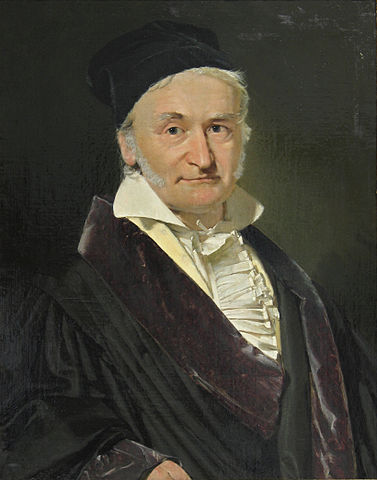# A useless non-RIP Gaussian matrixRecently, for some unrelated reasons, I discovered that it is actually very easy to generate a Gaussian matrix $$\Phi$$ that does not respect the restricted isometry property (RIP) . I recall that such a matrix is RIP if there exists a (restricted isometry) constant $$0<\delta<1$$ such that, for any $$K$$-sparse vector $$w \in \mathbb R^N$$, $(1-\delta)\|w\|^2\leq \|\Phi w\|^2 \leq (1+\delta)\|w\|^2.$

This is maybe obvious and it probably serves to nothing but here is anyway the argument.

Take a $$K$$-sparse vector $$x$$ in $$\mathbb R$$ and generate randomly two Gaussian matrices $$U$$ and $$V$$ in $$\mathbb R^{M\times N}$$ with i.i.d. entries drawn from $$\mathcal N(0,1)$$. From the vectors $$a=Ux$$ and $$b=Vx$$, you can form two new vectors $$c$$ and $$s$$ such that $$c_i = a_i / \sqrt{a_i^2 + b_i^2}$$ and $$s_i = b_i / \sqrt{a_i^2 + b_i^2}$$.

Then, it is easy to show that matrix $\Phi = {\rm diag}( c) V - {\rm diag}(s)U$ is actually Gaussian except in the direction of $$x$$ (where it vanishes to 0).

This can be seen more clearly in the case where $$x = (1,0,\cdots,0)^\top$$. Then the first column of $$\Phi$$ is $$0$$ and the rest of the matrix is independent of $${\rm diag}( c)$$ and $${\rm diag}(s)$$. Conditionally to the value of these two diagonal matrices, this part of $$\Phi$$ is therefore Gaussian with each $$ij$$ entry ($$j\geq 2$$) of variance $$c_i^2 + s_i^2 = 1$$. Then, the condition can be removed by expectation rule to lead to the cdf of $$\Phi$$, and then to the pdf by differentiation, recovering the Gaussian distribution in the orthogonal space of $$x$$.

However, $$\Phi$$ cannot be RIP. First, obviously since $$\Phi x = 0$$ which shows, by construction, that at least one $$K$$-sparse vector (that is, $$x$$) is in the null space of $$\Phi$$. Second, by taking vectors in $$x + \Sigma_K = x + \{u: \|u\|_0 \leq K \}$$, we clearly have $$\|\Phi (x + u)\| = \|\Phi u\|$$ for any $$u \in \Sigma_K$$. Therefore, we can alway take the norm of $$u$$ sufficiently small so that $$\|\Phi (x + u)\|$$ is far from $$\|x + u\|$$.

Of course, for the space of $$K$$-sparse vectors orthogonal to $$x$$, the matrix is still RIP. It is easy to follow the argument above for proving that $$\Phi$$ is Gaussian in this space and then to use classical RIP proof .

All this is also very close from the “Cancel-then-Recover” strategy developed in . The only purpose of this post to prove the (useless) result that combining two Gaussian matrices as in (1) leads to a non-RIP matrix.

References:

•  Candes, Emmanuel J., and Terence Tao. “Decoding by linear programming.” Information Theory, IEEE Transactions on 51.12 (2005): 4203-4215.
•  Baraniuk, Richard, et al. “A simple proof of the restricted isometry property for random matrices.” Constructive Approximation 28.3 (2008): 253-263.
•  Davenport, Mark A., et al. “Signal processing with compressive measurements.” Selected Topics in Signal Processing, IEEE Journal of 4.2 (2010): 445-460.

Image credit: Carl Friedrich Gauss, 1777-1855. Source Wikipedia.